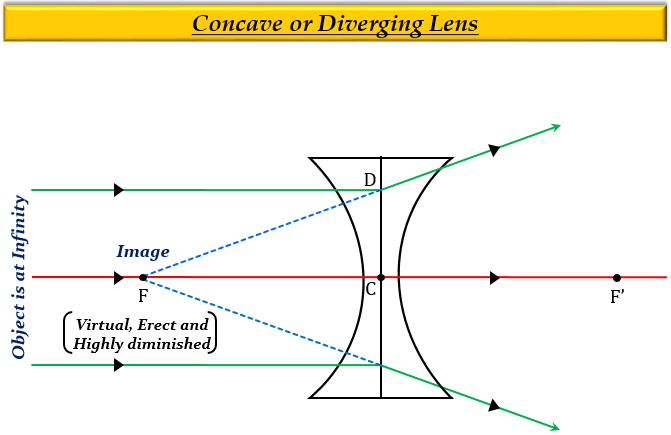# A 50 cm tall object is at a very large distance from a perging lens. A virtual, erect and diminished image of the object is formed at a distance of 20 cm in front of the lens. How much is the focal length of the lens?

When an object is placed at a very large distance (or, infinity) from a diverging lens, then the image will be formed at the focus $(F)$ of the lens. So, the image distance will be equal to the focal length $(f)$ of the lens, which is 20cm.

Explanation

For an object placed at infinity from a concave lens (diverging lens), the image is formed at the focus $(F)$, the nature of the image is virtual and erect, and the size is highly diminished.Updated on: 10-Oct-2022

120 Views